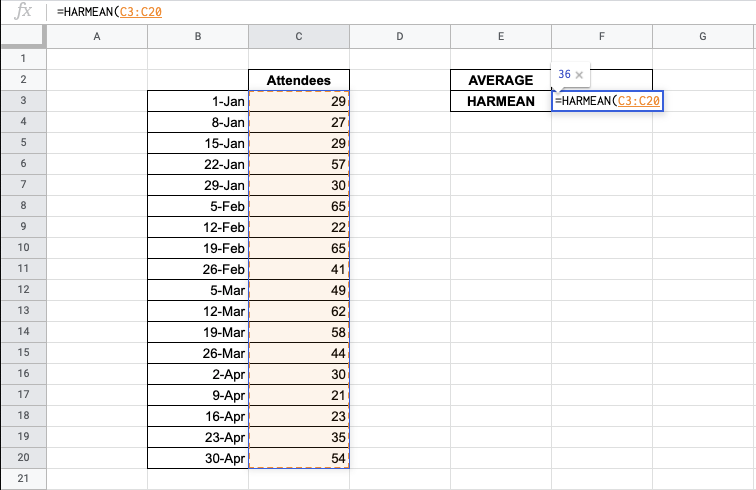# How to Use HARMEAN Function in Google Sheets

The HARMEAN function in Google Sheets is used to calculate the harmonic mean of a dataset.

The harmonic mean of a dataset is the size of the population divided by the sum of the reciprocal of each data point in the set. In other words, it’s the reciprocal of the mean of the reciprocals of a data set.

This function is useful because it helps you to find answers when you are dealing with ratios. It is used in the fields of physics, finance, geometry, and other sciences.

When might you use the harmonic mean, among all other types of averages and means? Let’s look at an example.

You are studying the different speeds that you and a friend are doing on your bike ride. For the first 10km, both of you were traveling at 20 kph. for the next 10 km, you decide to go faster at 30 kph. What was your average speed for the ride?

The `HARMEAN` function used in the calculation of the average of rates. This will give us the true answer to the question rather than just treating the speeds as plain numbers and taking their average.

## The Anatomy of HARMEAN Function in Google Sheets

The syntax of the `HARMEAN` function is as follows:

`=HARMEAN(value1, [value2, ...])`

Let’s have a look at each part of the function to understand what is going on here:

• `=` is the equals sign that starts off any function in Google Sheets.
• `HARMEAN` is the name of our function.
• `value1` is the first value or range of the dataset.
• `value2, ...` are the additional values or ranges to be included in the dataset.

Remember that when you are dealing with ratios or rates, you are likely needing to use `HARMEAN` over arithmetic averages.

## A Real Example of Using HARMEAN Function

You may be curious as to why the `HARMEAN` function exists, when there are other ways to calculate averages.

The `HARMEAN` function helps to create correct calculations with averaging rates and ratios. This gives an accurate answer and does well to remove large outliers on its own. This is helpful in a variety of very specific tasks.

Let’s look at the example below to see how to use the `HARMEAN` function in Google Sheets.

#### Calculating the average of rates in Google Sheets

This is a simple problem. It is a simplified task to help you understand what the function can be used for.In the equation, it will look like this:

```=HARMEAN(C3,C4)
```

It will result in an average of 24.

While the regular `AVERAGE` calculates it as 25, but this is not completely accurate. The `HARMEAN` takes note of this and calculates the average properly.

This simple problem can be practiced to perfection. Use the link below to get a copy of this problem set:

## How to Use HARMEAN Function in Google Sheets

In this section, we will show you a step-by-step process on how to use the `HARMEAN` function in Google Sheets.

Another feature of `HARMEAN` is to take of the outliers in a dataset.

In this problem, we are looking at a number of workshop attendees on certain days. We will find the harmonic mean of the attendees and compare it to the average.

#### Using HARMEAN Function In Google Sheets

1. To begin, prepare your spreadsheet with the information you want to check.1. Start to fill in the `HARMEAN` function in the cell you want it to appear. In this example, we pick cell F3.1. Start by adding in the `HARMEAN` function.1. Start by putting in the array of data you want to analyze. You will be able to see a preview.1. You’re done! But now, let’s compare to the regular average.1. You’ll notice that they have significantly different answers to offer, but the harmonic mean treats outliers in a more accurate manner.The `HARMEAN` function can help make accurate findings from your data.

And there you have it – you can now use the `HARMEAN` function in Google Sheets together with the other numerous Google Sheets formulas to create even more effective formulas.Our goal this year is to create lots of rich, bite-sized tutorials for Google Sheets users like you. If you liked this one, you'll love what we are working on! Readers receive ✨ early access ✨ to new content. There will be no spam and you can unsubscribe at any time.

##### You May Also Like## How to Sum Every N Cells to the Right or Bottom in Google Sheets

The circumstances of adding up every n cells in Google Sheets are countless. We may obsessively use the…## How to Use SPLIT Function in Google Sheets

The SPLIT function in Google Sheets is useful to quickly and easily separate text into columns based on…## How to Use NETWORKDAYS Function in Google Sheets

The NETWORKDAYS function in Google Sheets is useful if you want to know how many working days there…## How to Use IFERROR Function in Google Sheets

The IFERROR function in Google Sheets is used to effectively manage errors. Instead of showing an error message,…